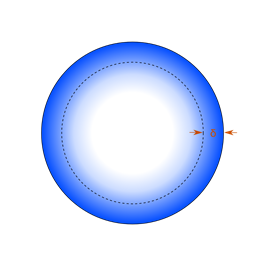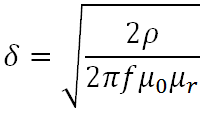#### Skin depth calculatorCross sectional distribution of current flow in a cylindrical conductor.

Skin depth is a measure of electrical conduction within a conductor. At DC (0 Hz) the current is equally distributed over the cross section of a conductor. As the frequency increases, the current distribution changes with the highest current density near the surface of the conductor.

Skin depth, δ, is a function of frequency, the conductor's relative permeability, μr, and resistivity, ρ (or conductivity, ο, where ο = 1/ ρ). It is defined as the depth below the surface of a conductor where the current density has fallen to 1/e (≈ 0.37) of the current density at the surface.Where

δ: Skin depth [m]

μr: Vacuum permeability [H.m-1]

ρ: Resistivity [Ω.m]

ο: Conductivity [S.m-1]